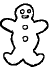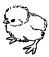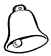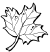Create a new printableMath Worksheets
Division

Sample - Click above to make a new math worksheet (PDF).
 Name _____________________________Date ___________________
Division
Complete.

1.
Divide 10 cookies into groups of 5.____ groups 10  ÷  5  =  ____
 2 * This is a pre-made sheet.Use the link at the top of the page for a printable page.
3.
Divide 12 birds into groups of 3.____ groups 12  ÷  3  =  ____
4.
Divide 12 bells into groups of 4.____ groups 12  ÷  4  =  ____
5.
Divide 12 leaves into groups of 4.____ groups 12  ÷  4  =  ____